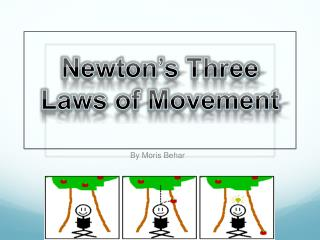Download PresentationNewton’s Three Laws of Movement

Newton’s Three Laws of Movement - PowerPoint PPT Presentation

Newton’s Three Laws of Movement. By Moris Behar . Newton’s Life Explained.I am the owner, or an agent authorized to act on behalf of the owner, of the copyrighted work described.
Download PresentationNewton’s Three Laws of Movement

Download Policy: Content on the Website is provided to you AS IS for your information and personal use and may not be sold / licensed / shared on other websites without getting consent from its author.While downloading, if for some reason you are not able to download a presentation, the publisher may have deleted the file from their server.

- - - - - - - - - - - - - - - - - - - - - - - - - - E N D - - - - - - - - - - - - - - - - - - - - - - - - - -
Presentation Transcript
1. Newton’s Three Laws of Movement By Moris Behar

2. Newton’s Life Explained Isaac Newton was presumed to be born on January 4th, 1643. He was born in England, and the British had not yet transferred to the Gregorian Calendar so a lot of people miswrote his birthdate. From age twelve to seventeen, Newton studied at “The King’s School” in Grantham. He then attended Trinity College and went off to study the law of gravitation.

3. Velocity and Acceleration To understand the First Law we must first understand what velocity and acceleration are. Velocity is speed in a certain direction. The formula for acceleration is the change in velocity over time.

4. First Law of Movement Now that we know what velocity and acceleration are, we need to know what resultant force means. Resultant force is the difference between the Newtons that are pushing an object forward and the Newtons that are pulling an object backward. So obviously if an object’s resultant force is big, then the object is fast. If the resultant force is a negative number, then the object is going backwards. Things that can pull an object back are air resistance and friction. Pushing an object forward can be caused by a slope, thrust, and the object’s own speed. When I pass a basketball to a teammate, the ball would slow down from the air resistance and be pulled down by gravity or its weight, but the thrust would make it go forward.

5. Quiz of First Law  Q1: What is velocity and acceleration? Q2: What is Newton’s First Law of Movement? Q3: What is the unit to describe the first law?

6. Answers to Quiz #1 • A1: Velocity is speed in a certain direction. Acceleration is the change over velocity over an amount of time. • A2: Newton’s First Law of Movement explains that the higher an object’s resultant force is, the faster it goes. • A3: The unit is N (Newton)

7. Second Law of Movement The second law is fairly simple. The equation for it is F = m x a (Force = mass x acceleration). So to figure out the force of an object you would need to use that equation. To find the mass you would need to divide the force by the acceleration. If my basketball’s mass is 6 kg and when I throw it is moving at 2 m/s squared, its force would be 12 N (F=m x a, F=6 x 2, F=12N).

8. Quiz of Second Law  • Q1: What is Newton’s Second Law of Movement? • Q2: What is the equation of the second law? • Q3: If a Mini Cooper has a mass of 700 kg and can accelerate at 6 miles per hour squared, what is its force?

9. Answers to Quiz #2 • A1: The second law of movement explains how to figure out an object’s force, mass, and acceleration. • A2: F = m x a (Force = mass x acceleration) • A3: 4,200 N

10. Third Law Of Movement Newton’s third law is about force being in pairs and it has to involve two objects. If I jump off a boat towards the dock, my legs push the boat back but they also help me go forward towards the shore. The two objects involved here are the boat and my legs. This happens because the boat and I have the same energy. “Every reaction has an equal and opposite reaction”.

11. Quiz of Third Law  • Q1: What is Newton’s Third Law of Movement about? • Q2: How many objects must there be for the third law to be valid?

12. Answers to Quiz #3 • A1: Newton’s third law explains how force comes in pairs. • A2: 2 objects

13. Conclusion Newton’s first law of motion explains the fact that the higher the resultant force of an object is the faster it goes. It also explains all the forces that move an object, forward or back. The second law of motion is F=m x a (Force=mass x acceleration). This tells us how to figure out an object’s force, mass, and acceleration. The third law of motion explains how force comes in pairs.